# Three excursions

Each pupil of the 9A class attended at least one of the three excursions. There could always be 15 pupils on each excursion. Seven participants of the first excursion also participated in the second, 8 participants of the first excursion, and 5 participants of the second excursion also attended the third. Four pupils participated at all three excursions. How many pupils were in the 9A class?

Result

n =  29

#### Solution:

$n_{1}=15-(8+7-4)=4 \ \\ n_{2}=15 - (7+5-4)=7 \ \\ n_{3}=15 - (8+5-4)=6 \ \\ \ \\ \ \\ n=n_{1}+n_{2}+n_{3}+(7+8+5-3 \cdot \ 4) + 4=4+7+6+(7+8+5-3 \cdot \ 4) + 4=29$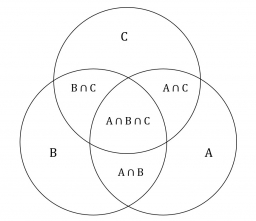Our examples were largely sent or created by pupils and students themselves. Therefore, we would be pleased if you could send us any errors you found, spelling mistakes, or rephasing the example. Thank you!

Leave us a comment of this math problem and its solution (i.e. if it is still somewhat unclear...):Be the first to comment!## Next similar math problems:

1. Mba studiumAt MBA school, fourth-year students can choose from three optional subjects: a) mathematical methods, b) social interaction, c) management Each student studies one of these subjects. The mathematical methods studied 28 students, the social interaction 27
2. Tunnels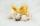Mice had built an underground house consisting of chambers and tunnels: • each tunnel leading from the chamber to the chamber (none is blind) • from each chamber lead just three tunnels into three distinct chambers, • from each chamber mice can get to an
3. Cows and calves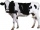There are 168 cows and calves in the cowshed. Cows are in nine stalls and calves in four stalls. Same count cows are in every cow stall and three more in each calf stall than in a cow stall. What is the capacity of the stalls for cows and what for calves?
4. Three daysDuring the three days sold in stationery 1490 workbooks. The first day sold about workbooks more than third day. The second day 190 workbooks sold less than third day. How many workbooks sold during each day?
5. Three friendsThe three friends spent 600 KC in a teahouse. Thomas paid twice as much as Paul. Paul a half less than Zdeněk. How many each paid?
6. Toy carsPavel has a collection of toy cars. He wanted to regroup them. But in the division of three, four, six, and eight, he was always one left. Only when he formed groups of seven, he divided everyone. How many toy cars have in the collection?
7. Summer camp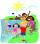Out of the 180 students at a summer camp, 72 signed up for canoeing. Twenty-three students signed up for trekking, and 13 of those students also signed up for canoeing. Use a two-way table to organize the information and answer the following question:
8. Three-digitHow many three-digit natural numbers do not have the number 7?
9. MushroomsEva and Jane collected 114 mushrooms together. Eve found twice as much as Jane. How many mushrooms found each of them?
10. The Chemistry testThe Chemistry test contained 8 questions, each with 3 points. Peter scored 21 points. How many percent did Peter write a test?In six baskets, the seller has fruit. In individual baskets, there are only apples or just pears with the following number of fruits: 5,6,12,14,23 and 29. "If I sell this basket," the salesman thinks, "then I will have just as many apples as a pear." Which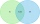Suppose Set B contains 69 elements and the total number elements in either Set A or Set B is 124. If the Sets A and B have 29 elements in common, how many elements are contained in set A?Mother is 39 years old. Her daughter is 15 years. For many years will mother be four times older than the daughter?In the orchard, they planted 25 apple trees, 20 pears, 15 plums and 40 marbles. A strong late frost, however, destroyed a fifth of all new trees. Unfortunately, it was all the trees of one kind of fruit. What is the probability that the plums have died ouIf you earned 80% of the possible 40 points, how many points did you miss to get 100%?x walnuts were in the mission. Dano took 1/4 of nuts Michael took 1/8 from the rest and John took 34 nuts. It stayed here 29 nuts. Determine the original number of nuts.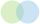How many 19 element's subsets can be made from the 26 element set?